# Mixed models with ciTools

## Introduction

Obtaining uncertainty estimates for prediction generated by mixed models (such as those fit by lme4) in R has been a challenge for users for many years. Unlike lm objections, there are no easy ways to get confidence intervals for predictions based on merMod objects, let alone prediction intervals. Options that did exist were based on simulation or bootstrapping and thus require considerable computation time. This tutorial describes the approach used to derive parametric intervals for random intercept models, how to generate them quickly and easily using ciTools, and provides discussion about how to appropriately interpret and use these intervals. A brief discussion of simulation results is provided at the end to show some properties of these intervals, including comparisons to bootstrap methods.

## Writing down our model

First, we’ll start by writing down our model and defining our terms so that we’re all on the same page. To an extent, I’ll follow the notation used by the author of lme4 (Doug Bates) in his 2010 book on the package.

$\textbf{Y}|\textbf{G} = \textbf{g} \sim N(X\beta + Z\textbf{g}, \sigma^2I)$ $\textbf{G} \sim N(0, \sigma_G^2I)$

In the above, $$\textbf{Y}$$ is our response vector for a particular group, $$X$$ is the design matrix containing fixed effects, $$\beta$$ is the vector of fixed effects parameters, $$Z$$ is the random effects matrix, $$\textbf{G}$$ is the vector of random effects with realizations $$\textbf{G}$$, $$\sigma^2$$ is the within-group variance, and $$\sigma_G^2$$ is the between-group variance, and $$I$$ is the identity matrix.

When considering uncertainty estimates for fitted values from this model, the following may be important sources of uncertainty:

1. $$\sigma^2$$
2. $$SE(\hat{\beta})$$
3. $$\sigma_G^2$$
4. $$SE(\hat{g})$$

Some of these are easier to estimate than others, and all of these can drive uncertainty when estimating both confidence intervals and prediction intervals in mixed models. We’ll refer to these sources of uncertainty below by the numbers associated with them in the above list.

## Types of intervals

### Conditional vs. unconditional confidence intervals

ciTools offers two approaches for estimating confidence and prediction intervals for mixed models, and they differ based on whether we want to condition on the random effects or not. We’ll look at the difference between conditional and unconditional intervals in terms of confidence intervals first. The “conditional” confidence interval is an interval for the expected value of an observation made under a particular set of covariate values conditional on that observation coming from a known group. In other words, this is a confidence interval for $$E(Y_{ij}|G_j = g_j)$$ for a particular value of $$g_j$$. (Note that while it would be more precise to state these expectations conditionally on a vector of covariates, $$x_{ij}$$ as well as $$g_j$$, we omit the covariate vector from the expression for simplicity.) When we estimate this conditional expectation, we have

$E(Y_{ij}|G_j=g_j) = E(X_{ij}\beta) + E(g_j) + E(\epsilon_{ij}) = X_{ij}\beta + g_j$

The standard error for our model estaimte is thus $$SE(\hat{Y}_{ij}) = SE(X_{ij}\hat{\beta}) + SE(\hat{g}_j)$$. Thus, a confidence interval for the group average will have a width determined by 2 and 4 from the above list.

Alternatively, we can consider an “unconditional” confidence interval for $$E(Y)$$. In this case, we are interested in the global average without conditioning on a particular group. Since $$E(G_j) = 0$$, we have $$\hat{Y}_{ij} = X_{ij}\hat{\beta}$$, and $$SE(\hat{Y}_{ij}) = SE(X_{ij}\hat{\beta})$$, which only includes 2 from the above list.

### Prediction intervals

Similarly, we can consider both conditional and unconditional prediction intervals. A $$1 - \alpha$$-level prediction interval for the $$i$$ observation from the $$j$$th group can be defined thus:

$(l, u) s.t. P(Y_{ij} \in (l, u)|G_j = g_j) = 1 - \alpha$

We can re-write this expression using the CDF for a Normal distribution,

$(l, u) s.t. \Phi(\frac{u-E(Y_{ij}|G_j = g_j)}{\sigma}) - \Phi(\frac{l-E(Y_{ij}|G_j = g_j)}{\sigma}) = 1- \alpha.$

Plugging in our estimated model parameters, we can find $$l$$ and $$u$$ by solving

$\Phi(\frac{\hat{u}- (X_{ij}\hat{\beta} + \hat{g_j})}{\hat{\sigma}}) = 1-\frac{\alpha}{2}.$

and

$\Phi(\frac{\hat{l}- (X_{ij}\hat{\beta} + \hat{g_j})}{\hat{\sigma}}) = \frac{\alpha}{2}.$

In this framework, 1, 2, and 4 are relevant sources of variance. Alternatively, consider the unconditional prediction interval:

$(l, u) s.t. P(Y \in (l, u)) = 1 - \alpha.$

$\Phi(\frac{\hat{u}- X_{ij}\hat{\beta}}{\hat{\sigma}_C}) = 1-\frac{\alpha}{2}$

and

$\Phi(\frac{\hat{l}- X_{ij}\hat{\beta}}{\hat{\sigma}_C}) = \frac{\alpha}{2},$

where $$\hat{\sigma}_C = \sqrt{\hat{\sigma}^2 + \hat{\sigma}^2_G}$$. For this unconditional prediction interval, 1, 2, and 3 determine our interval’s width.

## Interval demonstration

### Data

We’ll look at each of these intervals and compare their uses below. To do that, we need a data set. The code below generates a data set consisting of $$n_{groups} = 10$$ groups with $$n_{size} = 20$$ observations per group. The data set includes a two-level continous covariate and a continous covariate. The function x_gen_mermod generates the X-matrix, and y_gen_mermod takes this matrix and simualtes responses for given values of $$\sigma$$, $$\sigma_G$$, and $$\Delta$$, where $$\Delta$$ is the effect size of the factors. All of these values are set to a default of 1.

set.seed(20170812)
df <- x_gen_mermod(10, 20) %>%
y_gen_mermod()

Using this data set, we can fit a model using lme4::lmer to create an lmerMod object, which we will use for generating confidence and prediction intervals.

fit2 <- lmer(y ~ x1 * x2 + (1|group) , data = df)
fit2
## Linear mixed model fit by REML ['lmerMod']
## Formula: y ~ x1 * x2 + (1 | group)
##    Data: df
## REML criterion at convergence: 617.739
## Random effects:
##  Groups   Name        Std.Dev.
##  group    (Intercept) 0.9778
##  Residual             1.0582
## Number of obs: 200, groups:  group, 10
## Fixed Effects:
## (Intercept)          x1b           x2       x1b:x2
##      1.0536       1.2232       1.3286       0.4376

### Confidence intervals

The first interval we’ll look at is the conditional confidence interval. This is generated using add_ci and using the option includeRanef = TRUE. This option specifies that we want a conditional interval rather than unconditional. The default is includeRanef = FALSE, which will produce an unconditional interval. Recall from above that the conditional interval’s width is driven by both $$SE(\hat{\beta})$$ and $$SE(\hat{g})$$.

df %>% add_ci(fit2, type = "parametric", includeRanef = T) %>% head()
##   x1         x2 group          y    truth     pred  LCB0.025 UCB0.975
## 1  b 0.02152605     1 2.35197203 2.043052 2.686833 1.8179428 3.555723
## 2  a 0.26034694     1 1.67461276 1.260347 1.771473 0.9586722 2.584275
## 3  a 0.64673143     1 0.32537188 1.646731 2.284827 1.4908670 3.078787
## 4  a 0.69560555     1 0.01130084 1.695606 2.349762 1.5507213 3.148802
## 5  a 0.97354604     1 3.88958362 1.973546 2.719036 1.8614833 3.576589
## 6  b 0.15814781     1 3.12704262 2.316296 2.928137 2.0967721 3.759501

Plotting these results lets us visualize the uncertainty around our expected values. The figure below shows the parametric conditional confidence intervals described above for the first three groups from our example data set:

df %>%
filter(group %in% c(1:3)) %>%
add_ci(fit2, type = "parametric", includeRanef = T, names = c("LCB", "UCB")) %>%
ggplot(aes(x = x2)) +
geom_ribbon(aes(ymin = LCB, ymax = UCB), colour = "black", fill = "red1", alpha = 0.2) +
geom_line(aes(y = pred), colour = "red1", size = 2) +
facet_grid(x1 ~ group)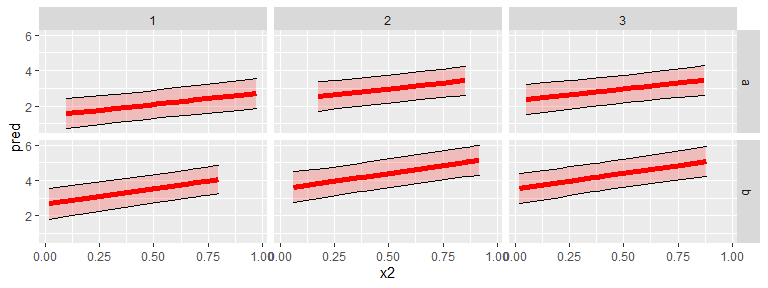For comparison, we consider bootstrap confidence intervals obtained by simulating from the fitted model using lme4::bootMer. For this function, the re.form = option is used to fit conditional (re.form = NULL) or unconditional (re.form = NA) intervals. (See ?lme4::bootMer for further information.) In ciTools, these intervals can be obtained by specifying type = boot.

set.seed(20170628)
df %>%
add_ci(fit2, includeRanef = T, type = "parametric", names = c("Lpar", "Upar")) %>%
add_ci(fit2, includeRanef = T, type = "boot", names = c("Lboot", "Uboot")) %>% head()
##   x1         x2 group          y    truth     pred      Lpar     Upar    Lboot
## 1  b 0.02152605     1 2.35197203 2.043052 2.686833 1.8179428 3.555723 2.071523
## 2  a 0.26034694     1 1.67461276 1.260347 1.771473 0.9586722 2.584275 1.246483
## 3  a 0.64673143     1 0.32537188 1.646731 2.284827 1.4908670 3.078787 1.739159
## 4  a 0.69560555     1 0.01130084 1.695606 2.349762 1.5507213 3.148802 1.784984
## 5  a 0.97354604     1 3.88958362 1.973546 2.719036 1.8614833 3.576589 2.075162
## 6  b 0.15814781     1 3.12704262 2.316296 2.928137 2.0967721 3.759501 2.353898
##      Uboot
## 1 3.292613
## 2 2.289008
## 3 2.762228
## 4 2.850508
## 5 3.320630
## 6 3.475644

We can plot our intervals side by side for comparison. The plot below shows both sets of conditional confidence intervals, with the intervals from simulate.merMod shown in red and the invervals from add_ci shown in blue.

set.seed(20170628)
df %>%
filter(group %in% c(1:3)) %>%
add_ci(fit2, includeRanef = T, type = "parametric", names = c("Lpar", "Upar")) %>%
add_ci(fit2, includeRanef = T, type = "boot", names = c("Lboot", "Uboot")) %>%
ggplot(aes(x = x2)) +
geom_ribbon(aes(ymin = Lpar, ymax = Upar), colour = "black", fill = "blue1", alpha = 0.2) +
geom_line(aes(y = pred), colour = "blue1", size = 2) +
geom_ribbon(aes(ymin = Lboot, ymax = Uboot), colour = "black", fill = "red1", alpha = 0.2) +
geom_line(aes(y = pred), colour = "red1", size = 2) +
facet_grid(x1 ~ group)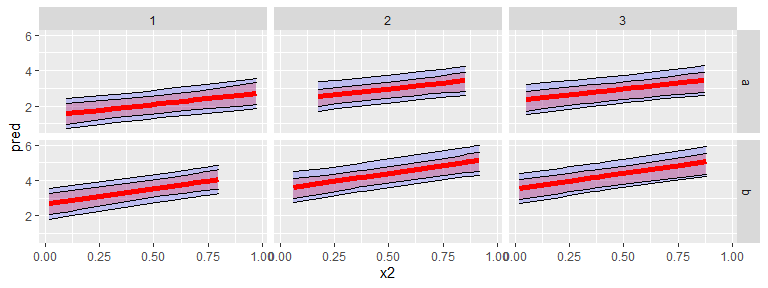Visually, these intervals look similar, with the parametric interval being wider than the bootstrap interval everywhere. The narrower width for the bootstrap approach comes at the expense of coverage probability, though as the number of groups and size of groups increase, these differences move towards zero. A later section will explore simulation results comparing these two approaches.

We can see that the results for unconditional intervals are also similar between the parametric and bootstrap methods. Unconditional intervals are generated add_ci by using the includeRanef = F option. Note that this is the default setting for includeRanef.

df %>% add_ci(fit2, includeRanef = F, type = "parametric") %>% head()
##   x1         x2 group          y    truth     pred  LCB0.025 UCB0.975
## 1  b 0.02152605     1 2.35197203 2.043052 2.314809 1.5737037 3.055914
## 2  a 0.26034694     1 1.67461276 1.260347 1.399449 0.7249772 2.073922
## 3  a 0.64673143     1 0.32537188 1.646731 1.912803 1.2611594 2.564447
## 4  a 0.69560555     1 0.01130084 1.695606 1.977738 1.3199136 2.635562
## 5  a 0.97354604     1 3.88958362 1.973546 2.347012 1.6192321 3.074792
## 6  b 0.15814781     1 3.12704262 2.316296 2.556113 1.8593819 3.252843

To illustrate the differences fitting the unconditional intervals vice the conditional intervals, the chart below shows the parametric unconditional intervals for a single group from our data set in blue while the conditional intervals as plotten in red.

set.seed(20170628)
df %>%
filter(group %in% c(1:3)) %>%
add_ci(fit2, includeRanef = F, type = "parametric", names = c("LU", "UU")) %>%
add_ci(fit2, includeRanef = T, type = "parametric", names = c("LC", "UC")) %>%
ggplot(aes(x = x2)) +
geom_ribbon(aes(ymin = LU, ymax = UU), colour = "black", fill = "blue1", alpha = 0.2) +
geom_ribbon(aes(ymin = LC, ymax = UC), colour = "black", fill = "red1", alpha = 0.2) +
facet_grid(x1 ~ group)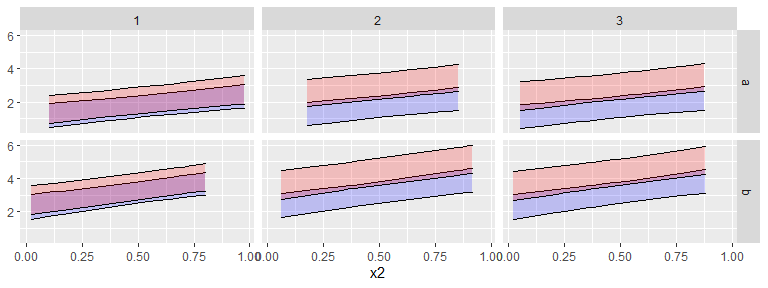The difference is readily apparent. For each group, the red intervals are shifted along the y-axis by the estimated group mean. Also note that the conditional intervals (red) are slightly wider than the unconditional intervals. This is due to the inclusion of the standard error for the group mean, which is unnecessary when estimating the unconditional group mean.

It’s also worth noting that the unconditional intervals for parametric and bootstrap methods are virtually identical, unlike the conditional intervals:

set.seed(20170628)
df %>%
filter(group %in% c(1:3)) %>%
add_ci(fit2, includeRanef = F, type = "parametric", names = c("Lpar", "Upar")) %>%
add_ci(fit2, includeRanef = F, type = "boot", names = c("Lboot", "Uboot")) %>%
ggplot(aes(x = x2)) +
geom_ribbon(aes(ymin = Lpar, ymax = Upar), colour = "black", fill = "blue1", alpha = 0.2) +
geom_line(aes(y = pred), colour = "blue1", size = 2) +
geom_ribbon(aes(ymin = Lboot, ymax = Uboot), colour = "black", fill = "red1", alpha = 0.2) +
geom_line(aes(y = pred), colour = "red1", size = 2) +
facet_grid(x1 ~ group)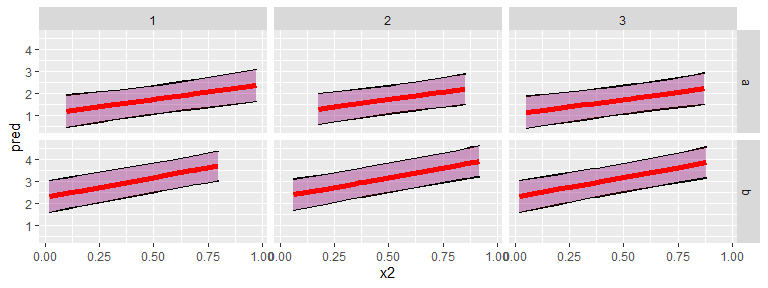Taking a step back, it’s not clear what exactly these interval estimates are useful for. The conditional estimates reflect fitted values for data that we do not expect to observe again. When we choose to treat a factor as random, it is because we are interested more in the magnitude of its variability rather than the specific values it takes within our sample. Therefore, the conditional mean estimates and their associated confidence intervals are not necessarily important One thing the conditional means may be useful for is visually demonstrating model fit. While not very interesting for future prediction, we can show visually the degree of model fit achieved by plotting the fitted values against these conditional mean estimates:

df %>%
add_ci(fit2, type = "parametric", includeRanef = T, names = c("LCB", "UCB")) %>%
filter(group %in% c(1:3)) %>%
ggplot(aes(x = x2)) +
geom_ribbon(aes(ymin = LCB, ymax = UCB), colour = "black", fill = "red1", alpha = 0.2) +
geom_line(aes(y = pred), colour = "red1", size = 2) +
geom_point(aes(y = y), size = 2) +
facet_grid(x1 ~ group)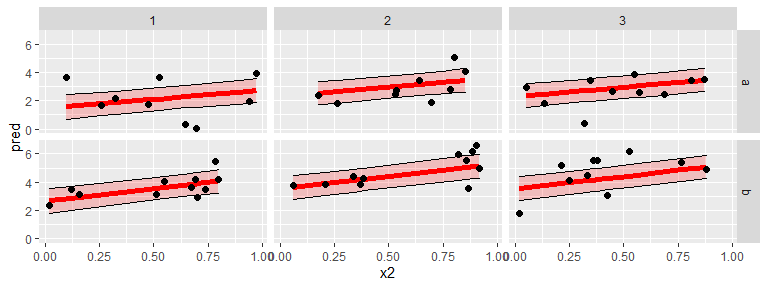Note that each “group” should be plotted seperately, even if multiple groups were observed under the same set of conditions. This is because the conditional interval estimates are conditional on a single group. The plot above shows data from groups 1, 2, and 3 by include group as a facet in facet_grid.

By way of contrast, attempting to plot fitted values against unconditional mean estimates could end up looking rather bad:

df %>%
add_ci(fit2, type = "parametric", includeRanef = F, names = c("LCB", "UCB")) %>%
filter(group %in% c(1:3)) %>%
ggplot(aes(x = x2)) +
geom_ribbon(aes(ymin = LCB, ymax = UCB), colour = "black", fill = "red1", alpha = 0.2) +
geom_line(aes(y = pred), colour = "red1", size = 2) +
geom_point(aes(y = y), size = 2) +
facet_grid(x1 ~ group)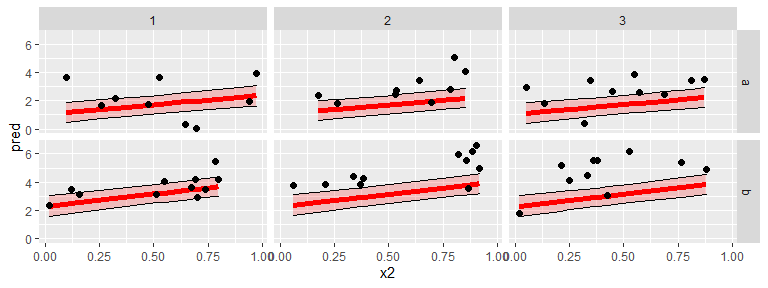The fact that these estimates fit poorly to the data is not a reflection of poor model fit but rather the construction of the unconditional intervals. These intervals are estimates for the expected value of each observation, unconditional on the group, explaining their divergence from the observed data. This is aside from the point that confidence intervals, such as those in the above plot, are measures of uncertainty about the estimate of the mean rather than measures of variance of the observed data around the mean.

A better way to use the unconditional confidence intervals would be to compare the response variable against some threshold value. If our response variable was some measure of performance, we may be interested in the conditions in which average system performance was better than some threshold value. The unconditional confidence intervals are useful when making such comparisons:

df %>%
add_ci(fit2, type = "parametric", includeRanef = F, names = c("LCB", "UCB")) %>%
ggplot(aes(x = x2)) +
geom_ribbon(aes(ymin = LCB, ymax = UCB), colour = "black", fill = "red1", alpha = 0.2) +
geom_line(aes(y = pred), colour = "blue", size = 2) +
facet_grid( ~ x1) +
geom_hline(yintercept = 1.5, colour = "red1", size = 2, linetype = 2, alpha = .5)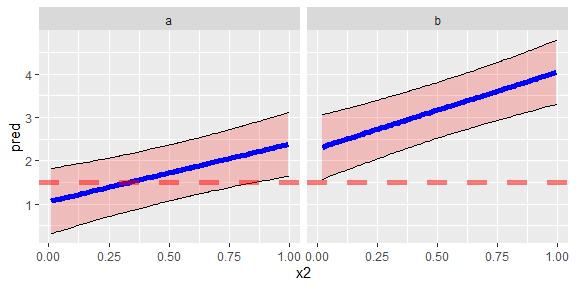From this plot, we can see the factor combinations where average system performance is better than the threshold value as well as those where it may not be.

It is inadviseable to include observed data on these plots, since the confidence bounds are estimates of the average and do not reflect either within-group or between-group variability.

### Prediction Intervals

Next, we will consider the conditional prediction interval. In addition to the parametric intervals described above an alternative approach is available using the type = "boot" option, which will estimate a prediction interval by simulating data from the fitted model using simulate.merMod.

Graphically, the prediction intervals should look like the conditional confidence intervals only wider:

df %>% add_pi(fit2, includeRanef = T, type = "parametric") %>% head()
##   x1         x2 group          y    truth     pred    LPB0.025 UPB0.975
## 1  b 0.02152605     1 2.35197203 2.043052 2.686833  0.42617699 4.947489
## 2  a 0.26034694     1 1.67461276 1.260347 1.771473 -0.46822327 4.011170
## 3  a 0.64673143     1 0.32537188 1.646731 2.284827  0.05189908 4.517755
## 4  a 0.69560555     1 0.01130084 1.695606 2.349762  0.11502223 4.584501
## 5  a 0.97354604     1 3.88958362 1.973546 2.719036  0.46271336 4.975359
## 6  b 0.15814781     1 3.12704262 2.316296 2.928137  0.68163659 5.174636
df %>%
filter(group %in% c(1:3)) %>%
add_ci(fit2, type = "parametric", includeRanef = T, names = c("LCB", "UCB")) %>%
add_pi(fit2, type = "parametric", includeRanef = T, names = c("LPB", "UPB")) %>%
ggplot(aes(x = x2)) +
geom_ribbon(aes(ymin = LCB, ymax = UCB), colour = "black", fill = "red1", alpha = 0.2) +
geom_ribbon(aes(ymin = LPB, ymax = UPB), colour = "black", fill = "blue1", alpha = 0.2) +
geom_line(aes(y = pred), colour = "red1", size = 2) +
facet_grid(x1 ~ group)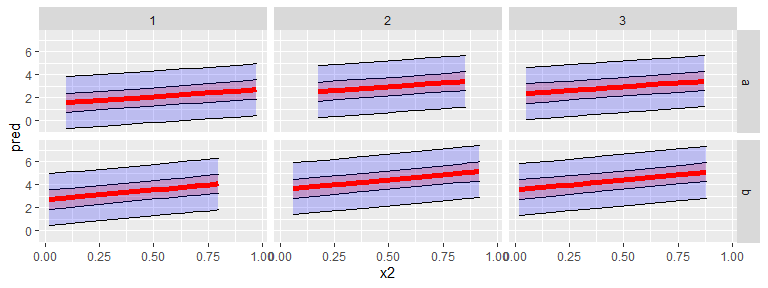Like the conditional confidence intervals, the best application for the conditional prediction intervals is showing model fit:

As above, each group should be plotted seperately, since these intervals do not account for between-group variance. Since we are now plotting prediction intervals, there should be no reservations about including the observed data on this plot.

Finally, the unconditional prediction interval:

df %>% add_pi(fit2, type = "parametric",includeRanef = F) %>% head()
##   x1         x2 group          y    truth     pred   LPB0.025 UPB0.975
## 1  b 0.02152605     1 2.35197203 2.043052 2.314809 -0.6218681 5.251486
## 2  a 0.26034694     1 1.67461276 1.260347 1.399449 -1.5211238 4.320023
## 3  a 0.64673143     1 0.32537188 1.646731 1.912803 -1.0025826 4.828189
## 4  a 0.69560555     1 0.01130084 1.695606 1.977738 -0.9390357 4.894511
## 5  a 0.97354604     1 3.88958362 1.973546 2.347012 -0.5863305 5.280355
## 6  b 0.15814781     1 3.12704262 2.316296 2.556113 -0.3696811 5.481906

In this case, there are a number of applications. Rather than comparing a mean value to a threshold, we are more likely to be interested in getting a visual indication of the likelihood that a single observation can meet or exceed a threshold:

df %>%
add_pi(fit2, type = "parametric", includeRanef = F, names = c("LPB", "UPB")) %>%
ggplot(aes(x = x2)) +
geom_ribbon(aes(ymin = LPB, ymax = UPB), colour = "black", fill = "blue1", alpha = 0.2) +
geom_line(aes(y = pred), colour = "red1", size = 2) +
geom_point(aes(y = y), size = 2) +
facet_grid( ~x1 )+
geom_hline(yintercept = 0, colour = "red1", size = 2, linetype = 2)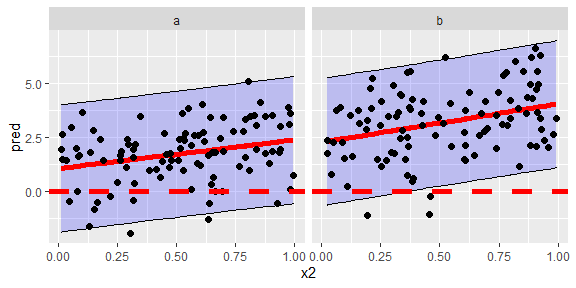In this case, there is no reason to include the groups as a facet. Since our estimates are not conditional on group, the estimates would be the same for each group. Therefore, we plot the full data set conditional on only the two factors, x1 and x2. Note that plotting fitted values may make sense in this case, as the estimated uncertainty bounds include both the between-group and within-group variability. This means that the intervals should contain the advertised percentage of the fitted values.

### Two intervals at once!

Finally, one potentially interesting visual could be to plot an overall prediction interval along with a single conditional confidence interval. This single plot would show both the overall variation for individual observations while also showing how a single group mean could drive variation:

df %>%
filter(group %in% c(1:3)) %>%
add_ci(fit2, type = "parametric", includeRanef = T, names = c("LCB", "UCB")) %>%
add_pi(fit2, type = "parametric", includeRanef = F, names = c("LPB", "UPB")) %>%
ggplot(aes(x = x2)) +
geom_ribbon(aes(ymin = LCB, ymax = UCB), colour = "black", fill = "red1", alpha = 0.2) +
geom_ribbon(aes(ymin = LPB, ymax = UPB), colour = "black", fill = "blue1", alpha = 0.2) +
geom_line(aes(y = pred), colour = "red1", size = 2) +
geom_point(aes(y = y), size = 2) +
facet_grid(x1 ~ group)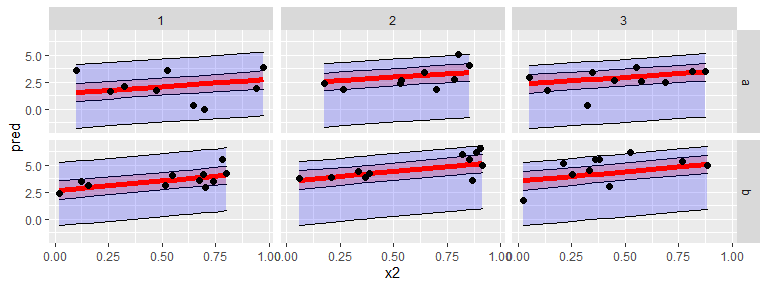This plot shows the unconditional prediction interval in blue along with confidence intervals conditional on group in red. The shifts in means between groups 1, 2, and 3 is clearly illustrated, with gorup 3 in particular having a large change in intercept due to group.

### add_probs and add_quantile

The other two primary functions in ciTools also have methods available for merMod objects. Mathematically, they follow the same arguemnts as the probability interval descritions given above, which we won’t repeat here. They follow the same conventions for conditioning (or not) on the random effects, and like the intervals discussed above, the choice of whether to condition or not should be made carefully and should depend on the intended use case. For population-level inference, unconditional probabilities and quantiles should be used, and these seem like the most common use cases.

## Simulation

To compare coverage probability and interval widths for intervals generated using the parametric and bootstrap methods described above, 1,000 data sets were generated for each combination of group size ($$n_{size} = 5, 10, 20, 50$$) and number of groups ($$n_{groups} = 5, 10 ,20, 50$$). Both methods were used to generate 80 percent confidence intervals for each data set. Random points within the prediction space were selected for each simulated data set to compare to the intervals for determining coverage probabilities.

### Unconditional confidence intervals

First, we consider the coverage probability of the unconditional parametric and bootstrap intervals. The blue line represnts the parametric intervals and hte red line the bootstrap intervals from ciTools. The x-axis denotes group size and the facet the number of groups in the data set.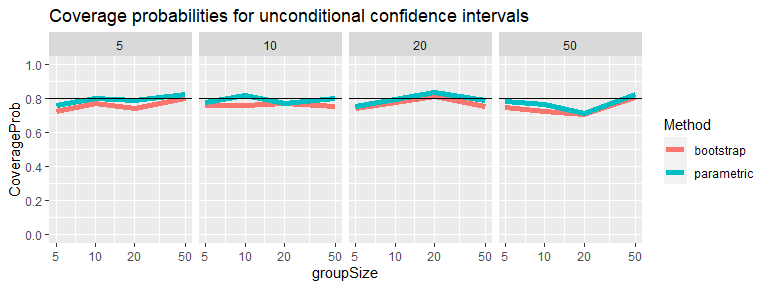We can see that these two approaches produce very similar results, with the parametric interval producing slightly higher coverage probabilities but both methods approaching their nominal level as the data set increases in size.Next we can consider the interval width. Once again, the two methods produce similar results. These results indicate that the unconditional partametric and bootstrap approaches perform similarly. The bootstrap is likely more robust to model misspecification (though this simulation did not explore this) but also takes considerably longer, particularly if you need to perform prediction across the complete design space. The parametric approach on the other hand takes virtually no computation time.

### Conditional confidence intervals

Unlike the unconditional intervals, the characteristics of conditional confidence intervals is not similar for bootstrap and parametric approaches. The first plot below reports average coverage probability on the y-axis, with the x-axis showing group size. The facet shows number of groups.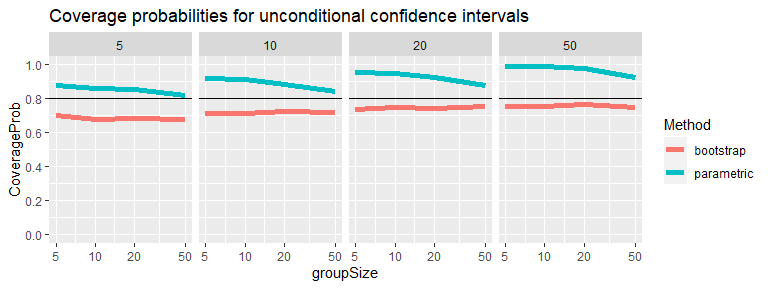The bootstrap method shows coverage probabilities below the nominal alpha level. As the number of groups increases, the coverage probability converges towards 80 percent, though increasing the group size does not appear to matter for coverage probability. In contrast, the parametric method shows coverage probabilities that exceed the nominal level. As group size increases, coverage probability coverages towards the nominal level, but when the number of groups increases, coverage probability increases towards 1. The former is an expected result of the standard errors for the $$X\beta$$ and the group means decreasing when the group sizes increase. The increase in coverage probability when group size increases is less easily explained. Particularly for cases when there are a large number of small groups, the parametric method produces extremely conservative interval estimates.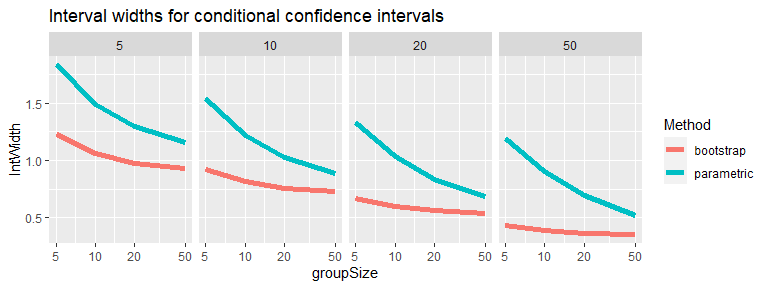These trends are also observable when we consider interval widths. The parametric intervals are substantially wider than the bootstrap intervals, though widths converge when the number of groups and group sizes increase.

In general, the more conservative parametric interval is recommended when there are few groups or at least the group size is large relative to the number of groups. When the group size is small relative to the number of groups, the parametric method available in ciTools is overly-conservative, and the bootstrap approach will provide more accurate coverage probabilities, although these will sitll not reach the nominal level.

### Unconditional prediction intervals

The approach for validating prediction intervals was slightly different than the approach for confidence intervals. The same sample sizes were used, but for determining coverage probability, one hundred test points were generated at five points in the design space for each randomly generated data set. Coverage probability was determined by calculating the proportion of these test points that were within the estimated prediction interval for both parametric and bootstrap methods.

Unconditional prediction intervals showed similar performance to unconditional confidence intervals in simulation.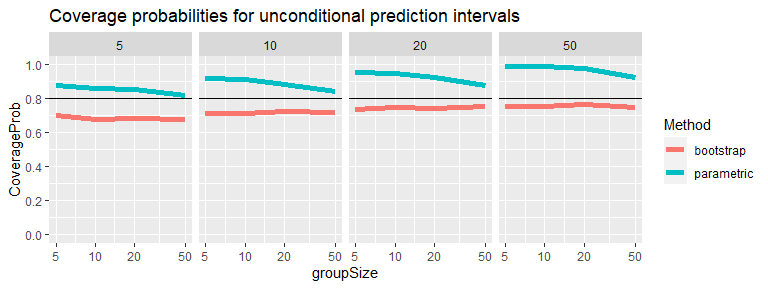As with the confidence intervals, coverage probability was slightly higher for the parametric method than the bootstrap, with the parametric method hewing closer to the nominal level, and the bootstrap interval falling just short. Neither interval’s coverage probabiltiy appears to be effected by the number of groups in the data set, though for small groups sizes, the parametric interval appears too wide for the nominal level.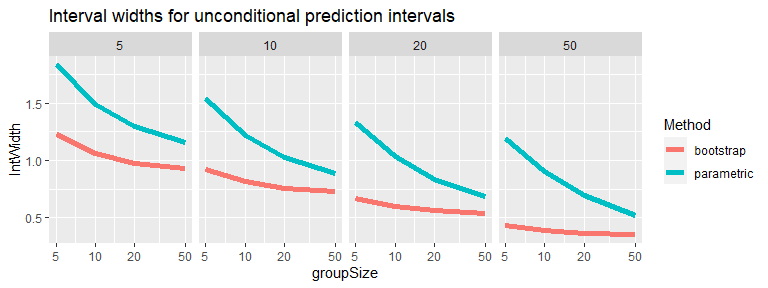As expected, the parametric intervals are wider than the bootstrap intervals. While the parametric interval width decreases with both the group size and number of groups, the bootstrap interval width increases as group size increases.

### Conditional prediction intervals

The performance of the bootstrap and parametric approaches is similar for conditional prediction intervals as it was for unconditional prediction intervals.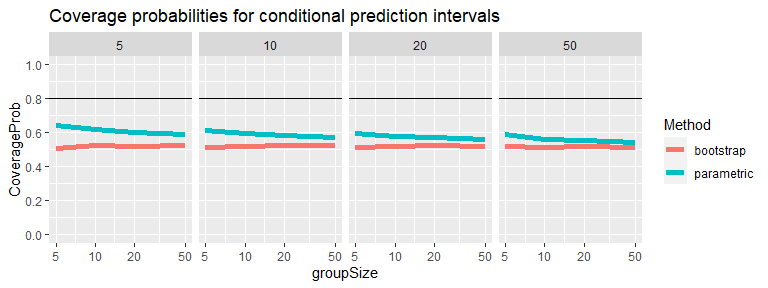Coverage probability for both intervals is near the nominal level, with the parametric approach producing wider intervals that are closer (though above) the nominal level. The bootstrap approach failes to reach the nominal coverage probability even for large sample sizes.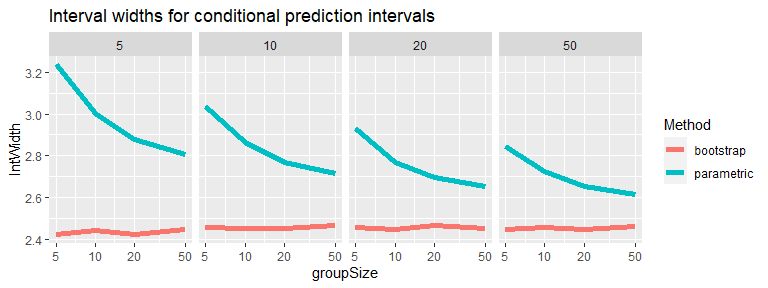Interval widths also show a similar pattern to the one shown for unconditional prediction intervals, with the parametric interval’s width converging towards the bootstrap interval’s width as group size and number of groups increases.